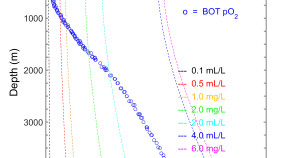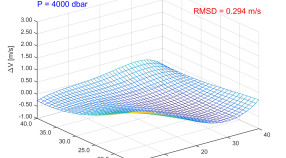### Matlab scripts: Linear regressions

Model I and Model II regressions are statistical techniques for fitting a line to a data set.### Matlab scripts: Oceanographic calculations

Calculate common oceanographic parameters (AOU, density, salinity, etc.) using scripts optimized for PCs.### Matlab scripts: Sound velocity

Calculate Vsnd (S,T,P) using a formula based upon the van ‘t Hoff equation.### Visual Basic for Excel: Oceanographic calculations

Calculate common oceanographic parameters (AOU, density, salinity, etc.) using scripts optimized for use with Excel.